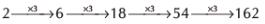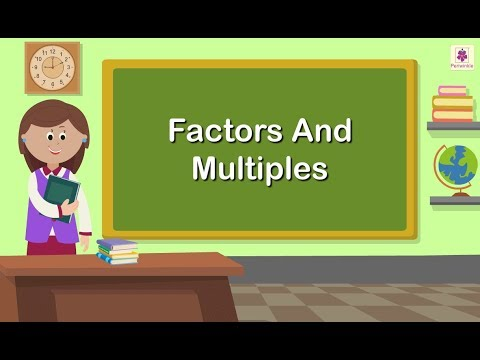Courses

# Olympiad Test: Factors And Multiples - 1

## 20 Questions MCQ Test Mathematics Olympiad Class 4 | Olympiad Test: Factors And Multiples - 1

Description
This mock test of Olympiad Test: Factors And Multiples - 1 for Class 4 helps you for every Class 4 entrance exam. This contains 20 Multiple Choice Questions for Class 4 Olympiad Test: Factors And Multiples - 1 (mcq) to study with solutions a complete question bank. The solved questions answers in this Olympiad Test: Factors And Multiples - 1 quiz give you a good mix of easy questions and tough questions. Class 4 students definitely take this Olympiad Test: Factors And Multiples - 1 exercise for a better result in the exam. You can find other Olympiad Test: Factors And Multiples - 1 extra questions, long questions & short questions for Class 4 on EduRev as well by searching above.
QUESTION: 1

Solution:
QUESTION: 2

Solution:
QUESTION: 3

### _____________ : 9 ::  4 : 24.

Solution:

Clearly 5 is a factor of 45 and not a multiple of 3.

QUESTION: 4

Which one of the following is a factor of 45 and not a multiple of 3?

Solution:
QUESTION: 5

Which one of the following is a multiple of 2 but not a factor of 8?

Solution:
QUESTION: 6

Which is odd one out?

Solution:

26, 39 and 65 are multiples of 13.

QUESTION: 7

Which is odd one out?

Solution:

12, 24 and 18 are multiples of 6.

QUESTION: 8

What are the multiples of 16 between 40 and 90?

Solution:

Clearly 48,64 and 80 are multiples of 16 between 40 and 90.

QUESTION: 9

What is seventh multiple of 9?

Solution:

Seventh multiple of 9 = 7 × 9 = 63

QUESTION: 10

What is eleventh multiple of 6?

Solution:
QUESTION: 11

What is next number in the sequence?
2, 6, 18, 54, ….

Solution:

The given sequence isQUESTION: 12

You are thinking of a number that is multiple of 7 and 12. What is the smallest number that you can think of?

Solution:
QUESTION: 13

What is the greatest length that is used to measure 8 m, 6 m and 14 m exactly?

Solution:
QUESTION: 14

Which of the following numbers has the least number of factors?

Solution:

Factors of 66: 1, 2, 3, 6, 11, 22, 33, 66.
Factors of 106: 1, 2, 53, 106.
Factors of 78 are 1, 2, 3, 6, 13, 26, 39, and 78.
Factors of 110: 1, 2, 5, 10, 11, 22, 55, 110.

QUESTION: 15

Which number below is a factor of 12?

Solution:

Clearly 6 is a factor of 12

QUESTION: 16

Which number below is not a factor of 8?

Solution:

3 is not a factor of 8.

The factors are 1,2,4 and 8.

(8/1 = 8, 8/2 = 4, 8/8 =1).

QUESTION: 17

Which number below is a factor of 16?

Solution:
QUESTION: 18

Which number below is a factor of 21?

Solution:
QUESTION: 19

Which number below is a factor of 25?

Solution:

The number 25 is a composite number. Now let us find the prime factors of 25.

• The first step is to divide the number 25 with the smallest prime factor,i.e. 2

25 ÷ 2 = 12.5

Therefore, 2 cannot be a prime factor of 25.

• Now, proceed to the next prime numbers, i.e. 3

25 ÷ 3 = 8.33

Therefore, 3 cannot be a prime factor of 25.

• Again, moving to the next prime number, 5 and continue dividing till you get fraction or 1 as the output.

25 ÷ 5 = 5

5 ÷ 5 = 1

• We have received 1 at the end in the division process and further, we cannot proceed. So, the prime factors of 25 are 5 × 5 or 52, where 5 is a prime number
QUESTION: 20

Which number below is not a factor of 20?

Solution:

Track your progress, build streaks, highlight & save important lessons and more!

### Similar Content### Related tests